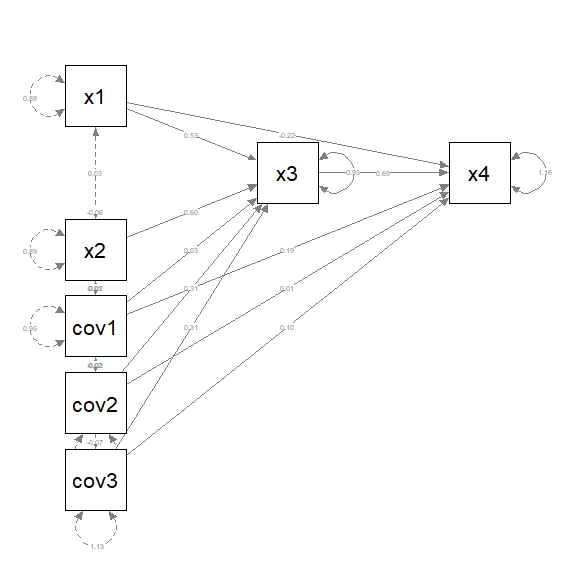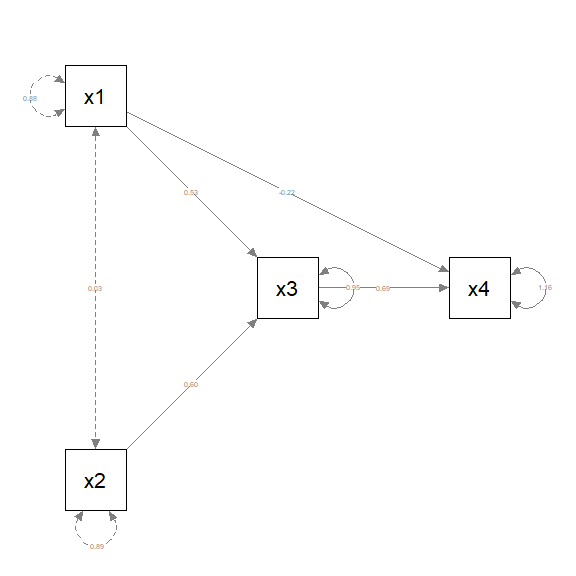# Introduction

In psychological research, it is common for a path analytic model to have several control variables. They need to be included in the analysis. However, for generating the plot, it is acceptable to omit them as long as results regarding these control variables are reported in other forms (e.g., a table of all parameter estimates), and keep only the main variables in the plot.

Two helper functions, drop_nodes and keep_nodes, were developed for this purpose. When an SEM output object, such as the output from lavaan, is passed to semPlot::semPaths, it is first processed by semPlot::semPlotModel. In other words, semPlot::semPaths itself does not interpret the SEM output. It actually use the output of semPlot::semPlotModel to generate the plot. These helper functions modify the output of semPlot::semPlotModel to be used by semPlot::semPaths.

# Example

Suppose we have a model with four main variables, x1, x2, x3, and x4, and three control variables, cov1, cov2, and cov3. The sample data, pa_example_3covs, is in the package semptools.

This is the analysis in lavaan:

library(lavaan)
#> This is lavaan 0.6-9
#> lavaan is FREE software! Please report any bugs.
mod <-
'x3 ~  x1 + x2 + cov1 + cov2 + cov3
x4 ~  x1 + x3 + cov1 + cov2 + cov3
'
fit <- lavaan::sem(mod, pa_example_3covs)

We can plot the full model using semPlot::semPaths and use layout and layout_matrix to control the positions of all seven variables (please refer to the vignette on layou_matrix on how to do this):

library(semPlot)
library(semptools)
m <- layout_matrix(x1 = c(1, 1),
x2 = c(3, 1),
x3 = c(2, 2),
x4 = c(2, 3),
cov1 = c(4, 1),
cov2 = c(5, 1),
cov3 = c(6, 1))
p_pa <- semPaths(fit, whatLabels = "est",
sizeMan = 10,
edge.label.cex = .5,
style = "ram",
nCharNodes = 0, nCharEdges = 0,
layout = m)Suppose we want to remove cov1, cov2, and cov3. We do this by calling semPlot::semPlotModel directly, and modify it by keep_nodes or drop_nodes.

We can drop cov1, cov2, and cov3 by drop_nodes:

pm_no_covs <- semptools::drop_nodes(
object = semPlotModel(fit),
nodes = c("cov1", "cov2", "cov3"))

The first argument, object, should be the output of semPlot::semPlotModel. In the example, semPlotModel(fit) is used to call semPlot::semPlotModel to process fit and then pass the results immediately to drop_nodes.

The second argument, nodes, is a character vector with the names of the variables to be dropped.

With just two arguments, the argument names can be omitted:

pm_no_covs <- semptools::drop_nodes(
semPlotModel(fit),
c("cov1", "cov2", "cov3"))

We can then use semPlot::semPaths to plot this modified model:

m_no_covs <- layout_matrix(x1 = c(1, 1),
x2 = c(3, 1),
x3 = c(2, 2),
x4 = c(2, 3))
pa_no_covs <- semPaths(pm_no_covs, whatLabels = "est",
sizeMan = 10,
edge.label.cex = .5,
style = "ram",
nCharNodes = 0, nCharEdges = 0,
layout = m_no_covs)Note that in the call to semPlot::semPaths, the modified output of semPlot::semPlotModel, pm_no_covs, is used instead of fit, the output of lavaan.

Alternatively, we can also specify the variables to keep using keep_nodes. It is used in a similar way, except that the variables specified in nodes will be kept, and all variables not specified will be removed.

pm_only_xs <- semptools::keep_nodes(
semPlotModel(fit),
c("x1", "x2", "x3", "x4"))
pa_only_xs <- semPaths(pm_only_xs, whatLabels = "est",
sizeMan = 10,
edge.label.cex = .5,
style = "ram",
nCharNodes = 0, nCharEdges = 0,
layout = m_no_covs)This plot is identical to the previous plot.

The plot generated by semPlot::semPaths with selected nodes dropped or kept can then be passed to other semptools functions for further processing.

# Keep Or Drop? It Depends

Which function to use depends on which one is easier to specify. For example, if there are a lot of contorl variables but only a few main variables, then it is more efficient to use keep_nodes and specify the few main variables. If the number of control variables is substantially less than the number of main variables, then it is more efficient to use drop_nodes and specify only the few control variables to drop.